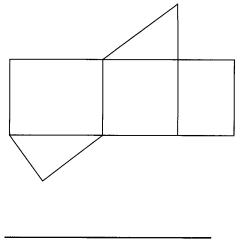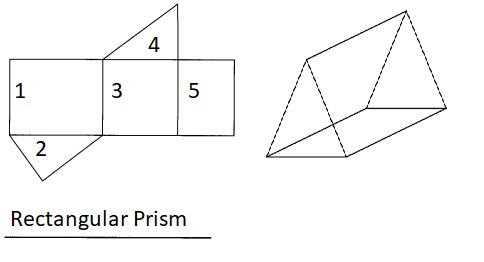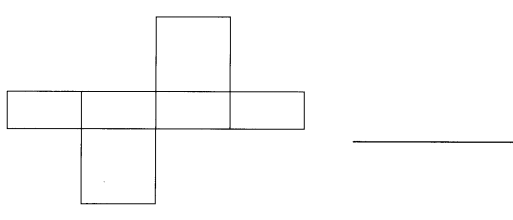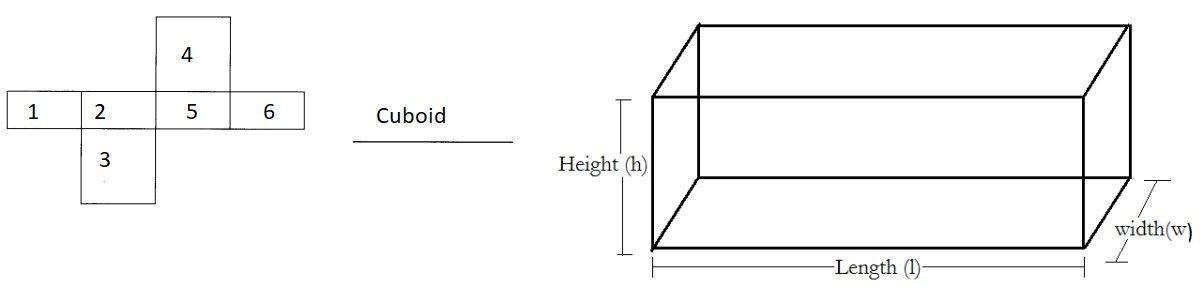# Math in Focus Grade 5 Chapter 14 Practice 1 Answer Key Prisms and Pyramids

This handy Math in Focus Grade 5 Workbook Answer Key Chapter 14 Practice 1 Prisms and Pyramids provides detailed solutions for the textbook questions.

## Math in Focus Grade 5 Chapter 14 Practice 1 Answer Key Prisms and Pyramids

Identify the type of pyramid and the shapes of the faces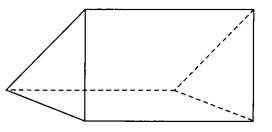Question 1.
This is a ___ prism.
This is a triangular prism,

Explanation:
A triangular prism is a prism composed of two triangular bases and
three rectangular sides.

Question 2.
Two of its faces are ____
Two of its faces are triangle,

Explanation:
A triangular prism is a prism composed of two triangular bases.

Question 3.
Three of its faces are ____
A triangular prism is a prism composed of three rectangular sides.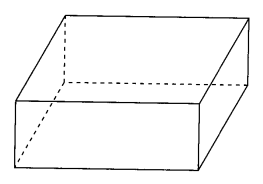Question 4.
This is a ____ prism.
This is a rectangular prism,

Explanation:
As a rectangular prism is a three-dimensional shape, having six faces,
where all the faces (top, bottom, and lateral faces) are rectangle of the prism.
So given solid is rectangular prism.

Question 5.
All its faces are _______________________
All its faces are rectangle,

Explanation:
As a rectangular prism is a three-dimensional shape, having six faces,
where all the faces (top, bottom, and lateral faces) are rectangle of the prism.

Complete the table.

Question 6.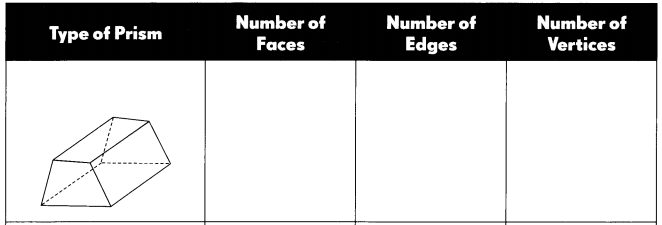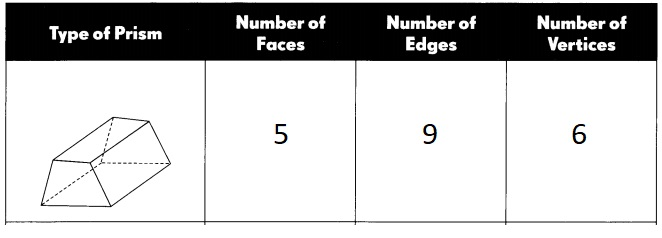Explanation:
Given type of prism is triangular as the prism is composed of two triangular bases and
three rectangular sides with number of faces – 5, number of edges – 9 & number of vertices – 6.

Question 7.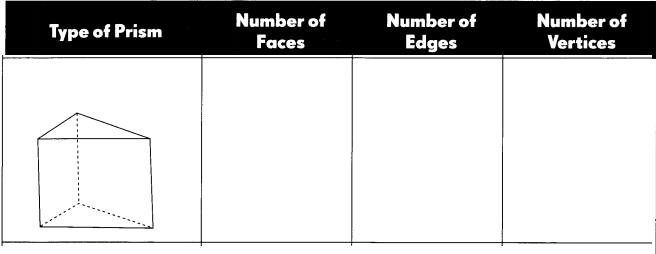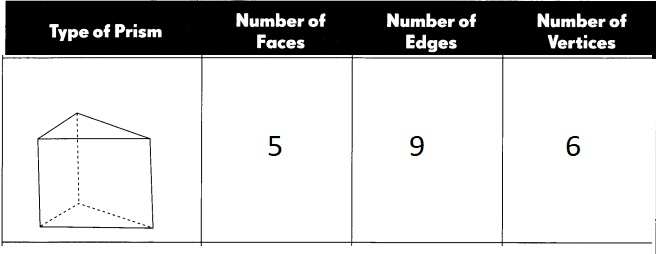Explanation:
Given type of prism is triangular as the prism is composed of two triangular bases and
three rectangular sides with number of faces – 5, number of edges – 9 & number of vertices – 6.

Question 8.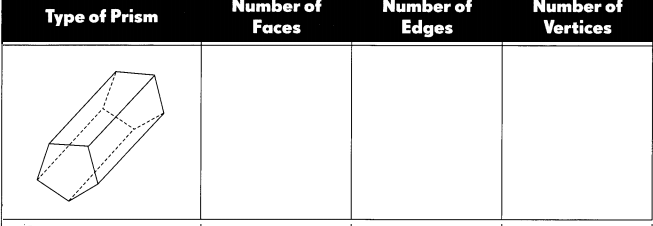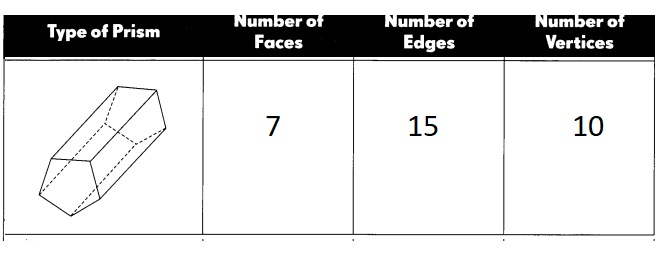Explanation:
Given a pentagonal prism is a three-dimensional solid that has two
pentagonal bases on the bottom and top of the shape.
All the other sides of a pentagonal prism have the shape of a rectangle.
There are types of pentagonal prism – regular pentagonal prism and
rectangular pentagonal prism.
A pentagonal prism has 7 faces, 15 edges and 10 vertices.

Identify the type of pyramid and the shape of the faces.

Question 9.
This is a ___ pyramid.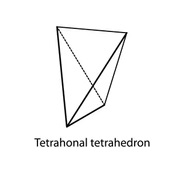Explanation:
Given pyramid is Tetragonal or Dipyramid with 4 prisms.

Question 10.
All its faces are __.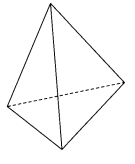All its faces are 8,

Explanation:
Given Tetragonal or Dipyramid has 8-faced form about a 4-fold rotation axis.

Identify the type of pyramid and the shapes of the faces.

Question 11.
This is a ___ pyramid.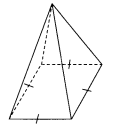This is a _tetragonal- dipyramid__ pyramid.

Explanation:
Given pyramid is Tetragonal or Dipyramid with 4 prisms.

Question 12.
One of its faces is a ____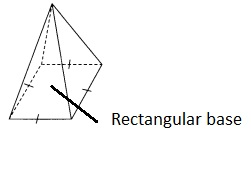One of its faces is a rectangular base,

Explanation:
Given pyramid has base of a rectangle as shown above.

Question 13.
Four of its faces are ____
Four of its faces are triangular prisms,

Explanation:
As given pyramid has four of its faces are triangular prisms.

Complete the table.

Question 14.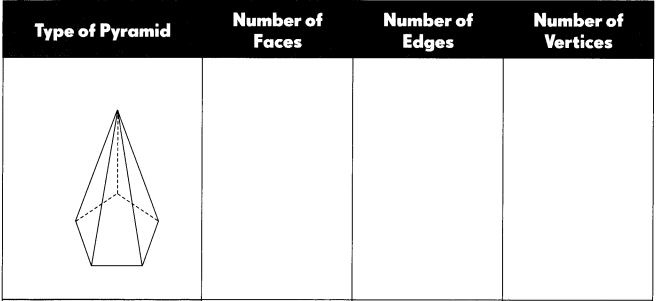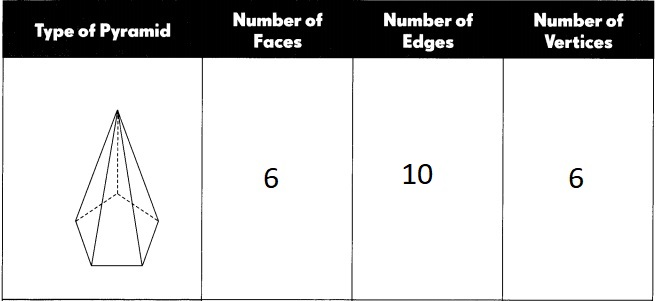Explanation:
Given type of pyramid is pentagonal pyramid number of faces 6, number of edges 10 and
number of vertices 6.

Question 15.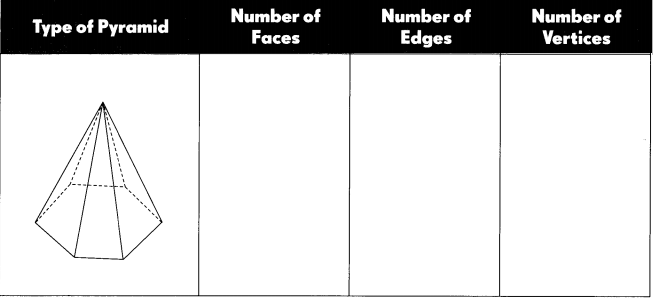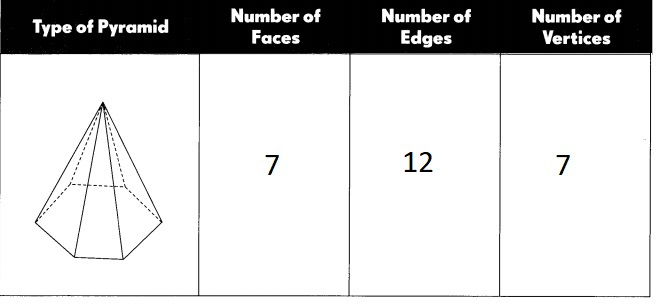Explanation:
Given the type of pyramid is a pentagonal pyramid number of faces 7,
a number of edges 12 and number of vertices 7.

Name the solid formed by each net.

Question 16.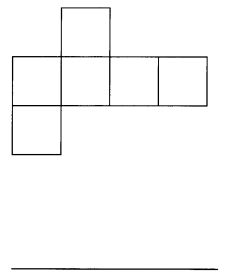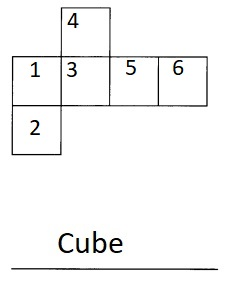Explanation:
Given net is Cube as as it has 6 square faces as shown above.

Question 17.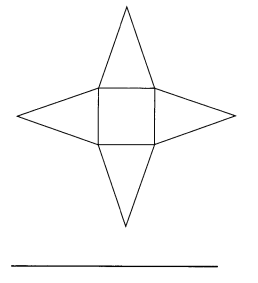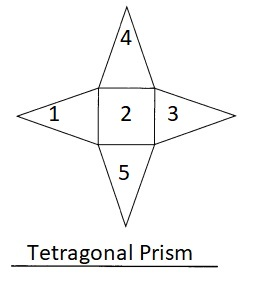Explanation:
Given net is tetragonal prism as as it has 4 triangular faces and one square face as shown above.

Question 18.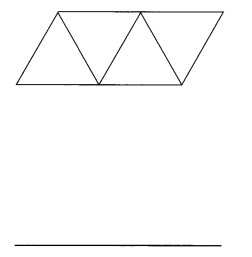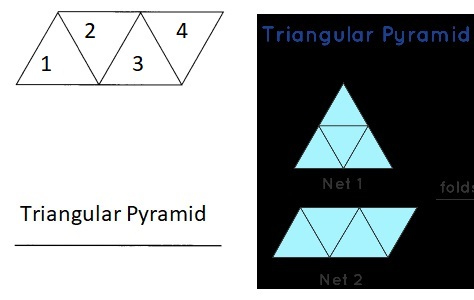Explanation:
Given net is triangular pyramid as as it has 4 triangular faces as shown above.

Question 19.